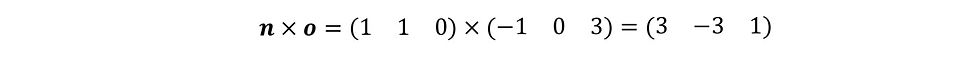top of page
Search
• Martin Scarratt

# Making Eigen CrossWhen you first encounter Eigenvectors and Eigenvalues you are told all about the Characteristic equation. I am not going to start any differently. Here is the standard introduction:When given a matrix you would replace A in the above formula with your matrix, find the determinant, set it equal to zero and solve the cubic (for a 3x3 matrix) polynomial that you are left with to find the three eigenvalues. I don't have a better way to do this.

When finding the eigenvectors you would usually substitute your eigenvalues into eqn (1) and attempt to solve the matrix equations that you form (which always turn out to be either one or two equations in three unknowns, giving an infinite number of solutions in each case), This isn't a great problem, but there is an interesting and more efficient method using the cross or vector product.That all sounds very complicated, maybe an example will show you how easy it is to actually do. You can come back to the explanation later once you are convinced of the simplicity of the method:First find the eigenvalues in the normal way (remember a triple scalar product will find you a determinant).Now we substitute these values into equation (1) the first being:Now the magic: simply cross two non-parallel row vectors to get your answerIt's as easy as that! Now do the same for the other eigenvalues:Any multiple of these final vectors is an eigenvector, so just multiply them by something 'nice' for your final answersIf you are proficient in the use of the cross product then this makes things somewhat quicker than the traditional method. Unfortunately it is only for 3x3 matrices, so it has its limitations. Now go back to the 'tricky' paragraph and see if you can understand why this works.